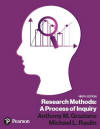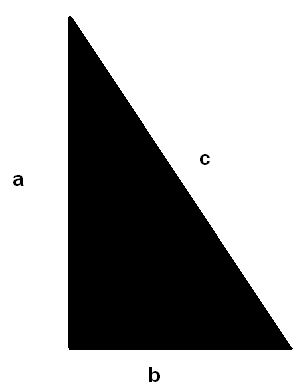﻿ Why Use the VarianceGraziano & Raulin
Research Methods (9th edition)

## Why Use the Variance

There are several predictable stumbling blocks for students in a statistics class, and the first one is usually the variance. It is not hard to learn how to compute it, but it difficult to understand. However, the most confusing aspect of the variance for most students is the question of why we use the variance in just about every other procedure that is covered in the course. That is is good question, and you deserve an answer that you can understand.

You probably learned the key to understanding why the variance is a useful statistic in high school if you took geometry. It has to do with the Pythagorean theorem. In case you do not remember this theorem off the top of your head, it says that the sum of the squares of the legs of a right triangle is equal to the square of the hypotenuse of the triangle. In case you have forgotten what some of those terms mean, a right triangle is a triangle that as a right angle (an angle that is 90°). The legs are the two sides that form that 90° angle, and the hypotenuse is the other side of the triangle. The figure below illustrates this situation and labels each of the sides of the triangle with a letter (a, b, and c).The Pythagorean theorem does NOT say that a + b = c. Instead, it says that a2 + b2 = c2. In other words, squared distances in this situation add up, and variance is a squared quantity.

What is the situation in which squared distances are additive? In a triangle, it is when there is a right angle. When variables are at right angles to one another, statisticians say that they are orthogonal. What that means is that the variables are uncorrelated with one another. Without going into the statistical details, research designs are set up so that key variables in the statistical analyses will automatically be orthogonal to one another. You need not understand the details of how this is achieved, only that it is achieved. This permits the mathematical development of a wide range of statistical analyses, many of which you will learn about in this text.

 Use the Back Arrow Key on the Browser Program to Return